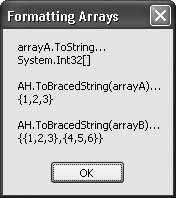# Recipe 8.13. Formatting an Array as a Single String

#### Problem

You want to format the contents of an array into a string, but the ToString() method returns only a string description of the array reference.

#### Solution

Sample code folder: Chapter 08 \PrintArrays

Build generic helper routines that format the contents of an array nicely.

#### Discussion

The ToString() method all objects inherit from System.Object is ideal in most cases; it gives you a quick and simple string representation of any object's contents. However with arrays, the ToString() method returns a description of the array, rather than a listing of its contents. This makes sense in that the array variable name contains a reference, not data, but it makes it tricky to get a listing of the array's contents.

The following code demonstrates how to format the contents of both one-and two-dimensional arrays generically. The two ToBracedString() functions accept an appropriately sized array and return a string with braces surrounding the array elements. The braces, data items, and separating commas are formatted in the same way as required when initializing an array in code. For example, output from this function for a two-dimensional array will have nested braces to indicate the layout of the array's rows and columns.

Here are the ToBracedString() functions used to display one-and two-dimensional arrays:

` Public Function ToBracedString(Of T)(ByVal sourceArray( ) _       As T) As String    ' ----- Display the contents of a one-dimensional array.    Dim result As New System.Text.StringBuilder    Dim counter As Integer    result.Append("{")    For counter = 0 To sourceArray.Length - 1       result.Append(sourceArray(counter).ToString( ))       If (counter < (sourceArray.Length - 1)) Then _          result.Append(",")    Next counter    result.Append("}")    Return result.ToString( ) End Function Public Function ToBracedString(Of T)(ByVal sourceArray(,) _   As T) As String    ' ----- Display the contents of a two-dimensional array.    Dim result As New System.Text.StringBuilder    Dim counter1 As Integer    Dim counter2 As Integer    Dim rank1Size As Integer = sourceArray.GetLength(0)    Dim rank2Size As Integer = sourceArray.GetLength(1)    result.Append("{")    For counter1 = 0 To sourceArray.GetLength(0) - 1       result.Append("{")       For counter2 = 0 To rank2Size - 1          result.Append(sourceArray(counter1, _             counter2).ToString( ))          If (counter2 < (rank2Size - 1)) Then _             result.Append(",")       Next counter2       result.Append("}")       If (counter1 < (rank1Size - 1)) Then result.Append(",")    Next counter1    result.Append("}")    Return result.ToString( ) End Function `

In the following code, two arrays are created and initialized with sample data, and their contents, as returned by ToBracedString(), are displayed for review:

` Dim result As New System.Text.StringBuilder Dim arrayA( ) As Integer = {1, 2, 3} Dim arrayB(,) As Integer = {{1, 2, 3}, {4, 5, 6}} ' ----- Show the typical ToString results. result.AppendLine("arrayA.ToString… ") result.AppendLine(arrayA.ToString) result.AppendLine( ) ' ----- Format arrayA nicely. result.AppendLine("ToBracedString(arrayA)… ") result.AppendLine(ToBracedString(Of Integer)(arrayA)) result.AppendLine( ) ' ----- Format arrayB nicely. result.AppendLine("ToBracedString(arrayB)… ") result.Append(ToBracedString(Of Integer)(arrayB)) MsgBox(result.ToString( )) `

Compare the braced initialization strings in the first few lines of the previous code with the output as shown in Figure 8-13. The goal was to duplicate the same simple format.

##### Figure 8-13. Using the ToBracedString() functions to format the contents of an arrayRecipe 8.1 shows how to properly format new array content in code.Visual Basic 2005 Cookbook: Solutions for VB 2005 Programmers (Cookbooks (OReilly))
ISBN: 0596101775
EAN: 2147483647
Year: 2006
Pages: 400

Similar book on Amazon